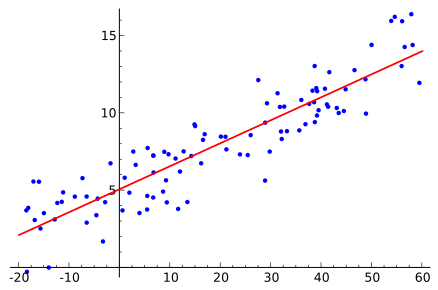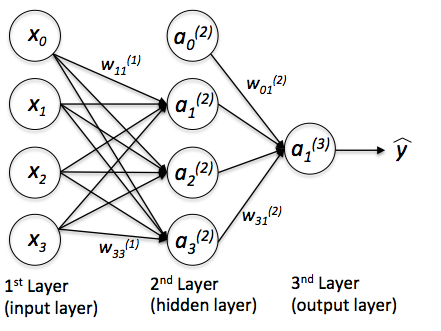# How I Learned to Stop Worrying and Love Uncertainty

This is a written version ofA graphical explanation of the p-value (Credit: Repapetilto & Chen-Pan Liao @ Wikipedia)

The p-value can be (inaccurately) thought of as the answer to the question “How likely would be the data I collected, given that my hypothesis was wrong?”, the idea being that if it is really small, then maybe the hypothesis is true. Clearly, the two questions are not the same, yet most p-value users equate them. To explain the exact nature of the error here, an important theorem in probability is needed, which I will discuss soon.

### Supervised Learning and its Limitations

A different approach can be taken, which assumes little to nothing about the nature of uncertainty and probability, and instead focuses its effort on producing the best prediction possible for a given task. This is the focus of supervised learning (SL), a type of machine learning (ML), which focuses on predicting a response variable given a set of input variables (AKA features) x, observed on a dataset.

Mathematically, SL algorithms are trying to estimate the expected value of the response variable given the input variables, as a function of them, by adjusting parameters through observations of these variables. Many powerful methods have been devised to perform this task, and one must choose among a diversity of them depending on the nature of variables, dimensionality, and complexity of the phenomena which produces the data, among other things.An example of an SL task, solved by Linear Regression (Credit: Sewaqu)

Because they are designed to do well on this problem, SL algorithms typically can’t deal with another type of questions. For example, one might wish to ask, given the input variables, how likely is it that the response rises above a given threshold. While this is a question that can be typically answered with a statistical model, not every ML model has a straightforward way to do it, and it is simply impossible for many.

Another problem that arises frequently when using some SL algorithms is the difficulty in interpreting their results. Take, for example, the multilayer perceptron, with many layers, an activation function per neuron (usually, per layer) and a lot of weights, it becomes quite difficult to explain what each parameter means, or pinpoint how a change in one of the inputs affects the response. Predictive power, in this case, comes at the cost of having to use the model as a sort of black box, its only task is to give out predictions, without context or interpretability.A multilayer perceptron (Credit: Sebastian Raschka)

When we use predictive models as black boxes, without being aware of the assumptions they make about the data and the phenomenon, we risk falling into overcertainty. Since we know our predictions to be accurate (it is not uncommon for an ML algorithm to rise above 90% accuracy), but we don’t exactly know the inner workings of them, we tend to trust them completely, much as if they were oracles, and make decisions taking for granted their predictions.

Some examples of the consequences of overcertainty:

• Google Flu Trends fails to “nowcast” the 2013 flu season
• Google labels a picture of black people as ‘Gorillas’
• A driver dies in fatal crash while trusting Tesla’s autopilot

A way to deal with overcertainty is to account for uncertainty, measuring and presenting it instead of reducing and hiding it, and a great framework to do this is known as Bayesian Statistics.